# Indoor Localization – An Introduction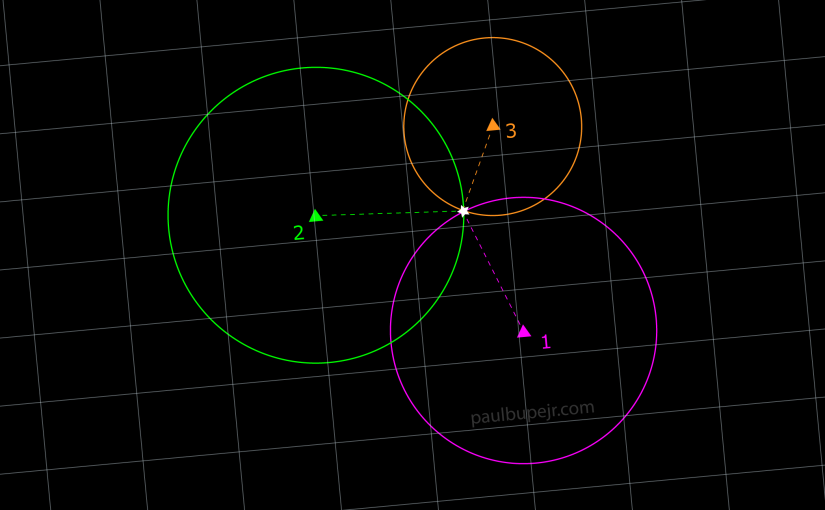Localization, in the context of robotics, is the process of determining the location of something within an environment. This article serves as a basic introduction to indoor localization, covering the commonly used techniques and technologies.

The most well known and widely used method of localization is GPS. Even with its widespread use, GPS is not suitable for all applications because it largely relies on line-of-sight (LOS) communication to GPS satellites. In indoor environments, these signals are greatly attenuated by walls and roofs, meaning that GPS often does not work indoors. Even when signals from at least four satellites are received indoors, the localization accuracy is too low to be useful. In lieu of GPS, a large number of indoor localization techniques and real-time locating systems (RTLS) have been developed. These can be grouped into two broad categories: radio-frequency (RF) based (wireless), and non-RF based (non-wireless).

Non-wireless techniques generally use cameras and sensors like Inertial Measurement Units (IMU) and laser distance finders (like LiDAR) for localization. Cameras can use markers or extract visual features from an environment to perform localization using various techniques including the very common technique known as simultaneous localization and mapping (SLAM). This technique involves building a map of an environment while at the same time localizing in that environment. Data from an IMU and laser scans can also be fused with the visual data to improve accuracy. These techniques are very computationally heavy, require specialized hardware, and are typically used in robotics or autonomous navigation applications (see Tesla, Boston Dynamics). This is the same type of localization technique I used in my last robot and I will cover this in more detail in a separate article.

Wireless techniques utilize technologies such as Wi-Fi, radio-frequency identification (RFID), Bluetooth, and ultra-wideband (UWB). These technologies are useful for indoor localization because they are already found in existing infrastructure and can be set up at very low cost. Wireless localization techniques can be classified as range-based, or range-free.

## Ranged-based Indoor Localization

Range-based (or distance-based) techniques are more accurate than range-free and involve measuring the distance from the unknown node being localized to some fixed nodes with known locations, typically called anchors. Most range-based localization algorithms utilize one of the common measurement techniques which fall into two categories: angle-based and distance-based. The most common angle-based measurement technique is Angle of Arrival (AoA) and the main distance measurement techniques are Time of Arrival (ToA), Time Difference of Arrival (TDoA) and Received Signal Strength (RSS).

#### Angle-based Measurement

AoA measurement techniques calculate the angle (bearing) between the unlocalized node and a fixed anchor with a known location. These measurements are obtained using two main techniques that include (1) measuring the amplitude response of the receiving antenna and (2) measuring the phase response of the receiving antenna. The location of the unknown node is a line having a certain angle from an anchor node — this requires at least two nodes to calculate the position as shown in the figure below.

The accuracy of these measurements is affected by the directivity of the antenna and the environmental NLOS and multipath effects. Since AoA measures angles, it requires direct LOS between the receiver and transmitter because a reflected signal arriving at the receiver can be interpreted as coming from a completely different direction, which can result in very large errors in the measurement.

#### Time of Arrival

Time of Arrival (ToA) is a technique that calculates distance based on the measured time of arrival of a signal from a transmitting node to a receiving one. This is more formally referred to as a one way propagation time measurement. The primary drawback to this technique is that it requires perfect time synchronization between the clocks of the transmitter and receiver nodes; any difference between the two clocks can become a large error in the distance calculation. Assuming normal conditions (air as the medium and radio waves traveling at the speed of light) a small clock synchronization error of 1ns will relate to a distance measurement error of 0.3m.

One way of overcoming this issue is by measuring the round trip propagation time instead of the one way propagation. The first node sends a signal to a second node which in turn immediately sends that signal back to the first node and the distance is calculated using the round trip time. This removes the need for the transmitter and receiver to have synchronized clocks. The primary issue with this round trip method is the processing delay accrued from the second node receiving the signal then sending it back in turn. This delay is usually known and specified by the manufacturer (or during a calibration process) so it can be subtracted from the measurement at the first node.

#### Time Difference of Arrival

Time Difference of Arrival (TDoA) is another technique that measures propagation time but in this case the difference between the arrival time of a signal at two different fixed receivers is measured. This requires that the location of the two receivers are known and those two receivers also have synchronized clocks. Unlike ToA, there is no need for the clocks between the transmitter and receivers to be in perfect sync.

There are two main methods of estimating distance using the Received Signal Strength (RSS): using the path loss log-normal shadowing model and RSS fingerprinting. Distance estimation using the path loss model is accomplished by measuring the signal attenuation as it propagates from the transmitting node to the receiving node. The relationship between distance and signal attenuation is heavily dependent on channel characteristics and as a result requires a very accurate propagation model in order to have acceptable results. The standard log-normal model used in this technique is as follows:

(1)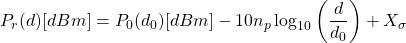with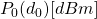being the reference power at distance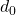from the transmitter,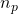being the path loss exponent measuring the rate at which the RSS decreases with distance, and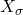being a zero mean Gaussian random variable with standard deviation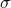which accounts for random showing effects.

### Multilateration

Multilateration is a core technique for estimating the location of a node using the measured distances to multiple anchors. Traditionally, this is achieved using 3 anchors (for 2D localization) and is referred to as trilateration. The locations of the anchors is assumed to be known and the location of the unknown node is the intersection of the three circles with the center at the location of each anchor and radius equal to the measured distance to the unknown node.

An example of the relationship between the node and anchors is shown in the figure above. In practice the measurements aren’t always accurate so the circles don’t intersect at a single point, as shown below.

In this case with the locations of the anchors and the estimated distances between the anchors and node known, trilateration then becomes a problem of solving three nonlinear circle equations

(2)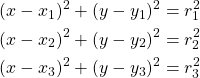where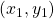,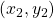, and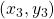, are the coordinates of anchors 1, 2, and 3. These equations can be linearized into the form

(3)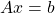with

(4)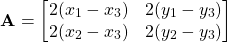(5)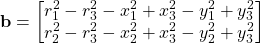(6)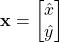which can be solved using the least squares method:

(7)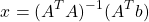Multilateration is not to be confused with triangulation which uses knowledge of the angles between the node and anchors to find the node-to-anchor distances using the law of sines.

## Range-Free Indoor Localization

Range-free techniques use the relative positions of existing nodes, connectivity information, or detecting the proximity of the unknown node to fixed anchors with known locations (using RFID or Bluetooth beacons) to localize. They are simpler, cheaper, and more energy efficient than range-based algorithms at the cost of having low localization accuracy. Because of this, range-free algorithms are generally only useful when coarse locations are desired.

#### Connectivity-based

Connectivity-based localization works by checking if a node is connected to another node. If each node is aware of all the connected nodes then coarse locations can be determined by counting the hops between nodes using an algorithm DV-Hop. Another technique is simply detecting the presence of a node near a knwon beacon, typically using RFID or Bluetooth.

#### Profiling / Fingerprinting

Another type of range free localization is the use of RSS profiling or fingerprinting in order to overcome the inaccuracies of RSS-based distance measurements. Fingerprinting involves taking RSS measurements at various locations and building a map of those measurements and their position. Localization then becomes a matter of matching RSS measurements, not distances, to the map in order to localize. This type of localization is very well suited for machine learning.

## Next Steps

This should hopefully have been a quick introduction to the concept of localization and the common techniques currently in use. The next article will include some code samples for implementing some of these techniques using Python.